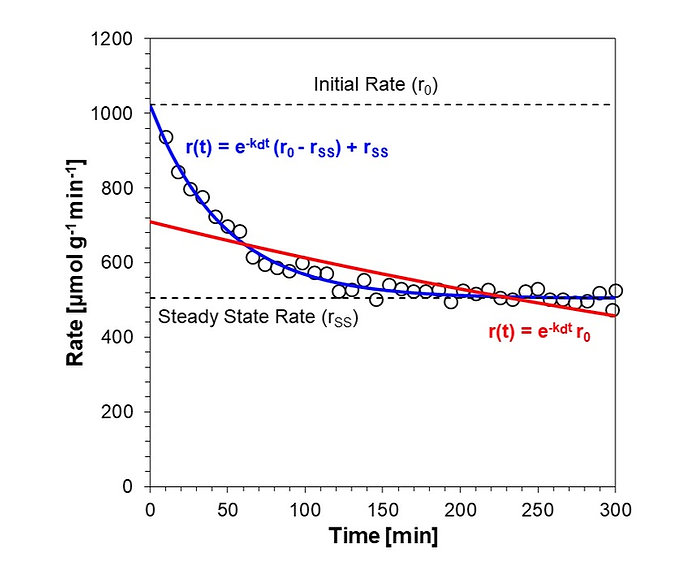## Temperature Programmed Desorption

Temperature programmed desorption (TPD) methods are powerful in deciphering underlying catalytic mechanisms and quantifying desorption energetics; interpreting the results of a TPD experiment is enhanced by being able to make predictions. Use the MATLAB code below to make TPD predictions based on the Polanyi-Wigner equation.TPD of tert-butylamine adsorbed on H-ZSM-5 with an activation entropy and enthalpy of 44 and 165 J/mol.K and kJ/mol, respectively.

## Peak Deconvolution

Signals from analytical instruments like gas chromatography don't always provide the needed time resolution to quantify independent peak contributions. Use the EXCEL file below to automatically deconvolute two overlapping peak signals, using Gaussian, Lorentzian or Voigt peak fitting. Note: Solver add-in required to use peak deconvolution tool.Deconvolution of two overlapping peaks in a GC-FID chromatogram

## Initial Rate Estimation

Creating structure function relationships by correlating material characteristics and their respective catalytic behavior is a hallmark of catalysis science. This requires the accurate measurement of reaction kinetics under conditions representative of the characterization method employed. For solid catalysts characterized using ex-situ techniques, an accurate measure of the initial rate of the catalyst on a pristine surface is needed. The files below help extract an intial rate estimate, that can be more readily compared to ex-situ characterization results. Both EXCEL and MATLAB files perform the same intial rate estimation, but the latter also provides confidence intervals on estimates.Comparison of two first order deactivation models for estimating an initial rate; zero vs non-zero catalytic activity at steady state